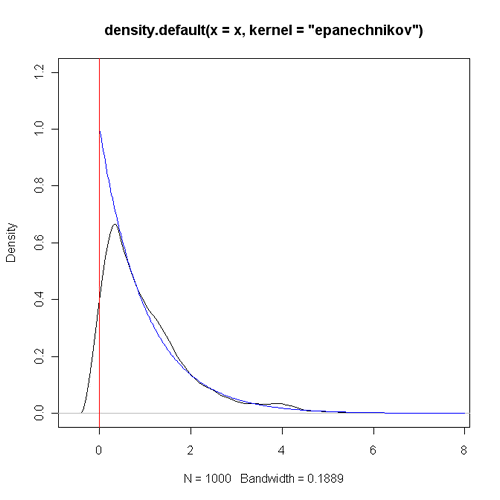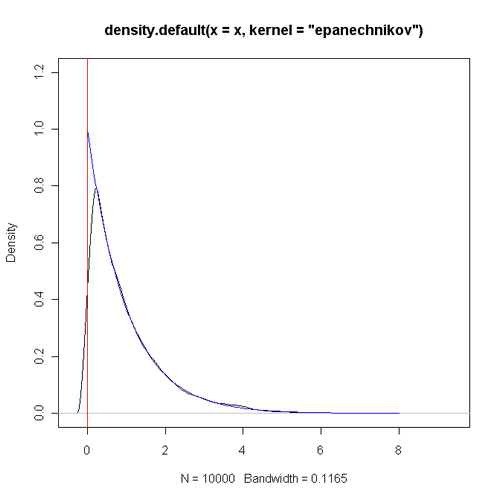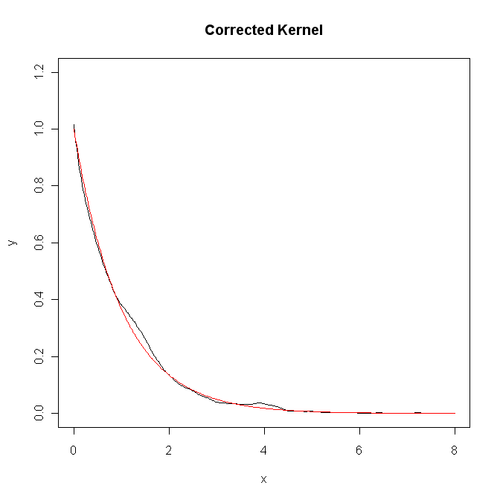# 一、一个例子

set.seed(123)
x <- rexp(1000, 1)
plot(density(x, kernel = "epanechnikov"), ylim = c(0, 1.2))
lines(seq(0, 8, by = 0.02), dexp(seq(0, 8, by = 0.02), 1), col = "blue")
abline(v = 0, col = "red")set.seed(123)
x <- rexp(10000, 1)
plot(density(x, kernel = "epanechnikov"), ylim = c(0, 1.2))
lines(seq(0, 8, by = 0.02), dexp(seq(0, 8, by = 0.02), 1), col = "blue")
abline(v = 0, col = "red")# 二、理论分析

$$E[\hat{f}_k(x)]=a_0(x)f(x)-ha_1(x)f'(x)+O(h^2)$$

$$E[\hat{f}_l(x)]=b_0(x)f(x)-hb_1(x)f'(x)+O(h^2)$$

$$b_1(x)*E[\hat{f}_k(x)]-a_1(x)*E[\hat{f}_l(x)]=[b_1(x)a_0(x)-a_1(x)b_0(x)]f(x)+O(h^2)$$

$$p(x)=\frac{b_1(x)k(x)-a_1(x)l(x)}{b_1(x)a_0(x)-a_1(x)b_0(x)}$$

$$p(x)=\frac{(a_2(x)-a_1(x)x)k(x)}{a_0(x)a_2(x)-a_1^2(x)}$$

$$x \ge h$$时，这个新的核函数$p(x)$就是$k(x)$，而当$$x \ge h$$时（也就是在边界），它会对最初的核函数进行调整。当$$x<0$$时，不管算出来的估计值是多少，都只需将密度的估计值取为0即可。

# 三、程序实现

k <- function(x) 3 / 4 * (1 - x^2) * (abs(x) <= 1)
a0 <- function(u, h) {
lb <- -1
ub <- pmin(u / h, 1)
0.75 * (ub - lb) - 0.25 * (ub^3 - lb^3)
}
a1 <- function(u, h) {
lb <- -1
ub <- pmin(u / h, 1)
3 / 8 * (ub^2 - lb^2) - 3 / 16 * (ub^4 - lb^4)
}
a2 <- function(u, h) {
lb <- -1
ub <- pmin(u / h, 1)
0.25 * (ub^3 - lb^3) - 0.15 * (ub^5 - lb^5)
}
kernel.new <- function(x, u, h) {
k(x) * (a2(u, h) - a1(u, h) * x) / (a0(u, h) * a2(u, h) - a1(u, h)^2)
}
den.est <- function(u, ui, h) {
sapply(u, function(u) ifelse(u < 0, 0, mean(kernel.new((u - ui) / h, u, h)) / h))
}
set.seed(123)
dat <- rexp(1000, 1)
x <- seq(0, 8, by = 0.02)
y <- den.est(x, dat, 2 * bw.nrd0(dat))
plot(x, y, type = "l", ylim = c(0, 1.2), main = "Corrected Kernel")
lines(x, dexp(x, 1), col = "red")# 四、参考文献

Jeffrey S. Simonoff, 1998. Smoothing Methods in Statistics. Springer-Verlag：http://pages.stern.nyu.edu/~jsimonof/SmoothMeth/